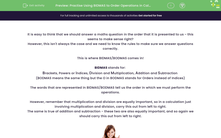# Practise Using BIDMAS to Order Operations in Calculations

In this worksheet, students will learn how to use the rules of BIDMA/BODMAS.Key stage:  KS 2

Curriculum topic:   Number: Addition, Subtraction, Multiplication and Division

Curriculum subtopic:   Carry Out Calculations Using Order of Operations

Difficulty level:#### Worksheet Overview

It is easy to think that we should answer a maths question in the order that it is presented to us - this seems to make sense right?

However, this isn't always the case and we need to know the rules to make sure we answer questions correctly.

This is where BIDMAS/BODMAS comes in!

BIDMAS stands for:

Brackets, Powers or Indices, Division and Multiplication, Addition and Subtraction

(BODMAS means the same thing but the O in BODMAS stands for Orders instead of Indices)

The words that are represented in BIDMAS/BODMAS tell us the order in which we must perform the operations.

However, remember that multiplication and division are equally important, so in a calculation just involving multiplication and division, carry this out from left to right.

The same is true of addition and subtraction - these two are also equally important, and so again we should carry this out from left to right.Examples

6 + 9 ÷ 3 = 6 + 3 = 9 (divide first)

(6 + 9) ÷ 3 = 15 ÷ 3 = 5 (brackets first)

15 - 4 × 2 = 15 - 8 = 7 (multiply first)

(15 - 4) × 2 = 11 × 2 = 22 (brackets first)

4 × 32 = 4 × 9 = 36 (power first)

(4 × 3)2 = 122 = 144 (brackets first)

14 - 4 + 5 = 15 (equally important, so left to right)

Now, why don't you have a go at answering some questions using BIDMAS.

Come back and have another look at this page if you get stuck by clicking on the red help button on the screen.Good luck!

### What is EdPlace?

We're your National Curriculum aligned online education content provider helping each child succeed in English, maths and science from year 1 to GCSE. With an EdPlace account you’ll be able to track and measure progress, helping each child achieve their best. We build confidence and attainment by personalising each child’s learning at a level that suits them.

Get started••••